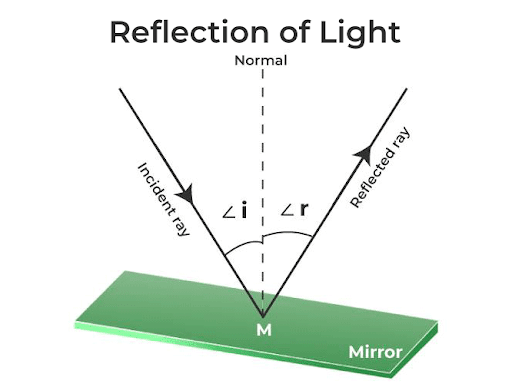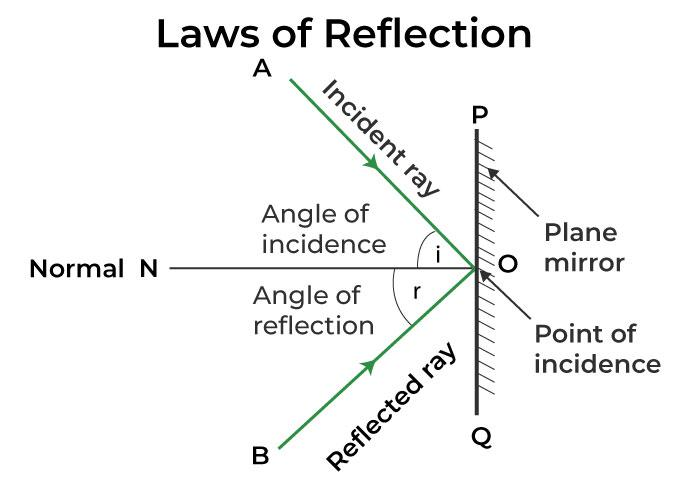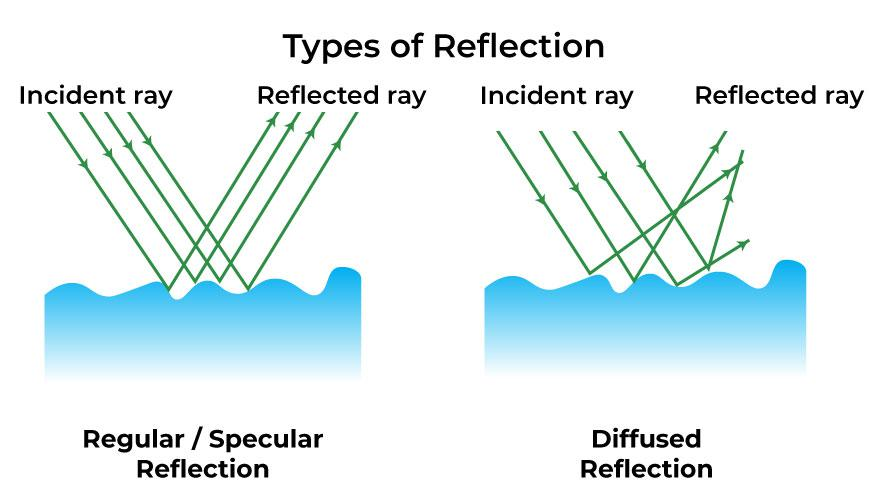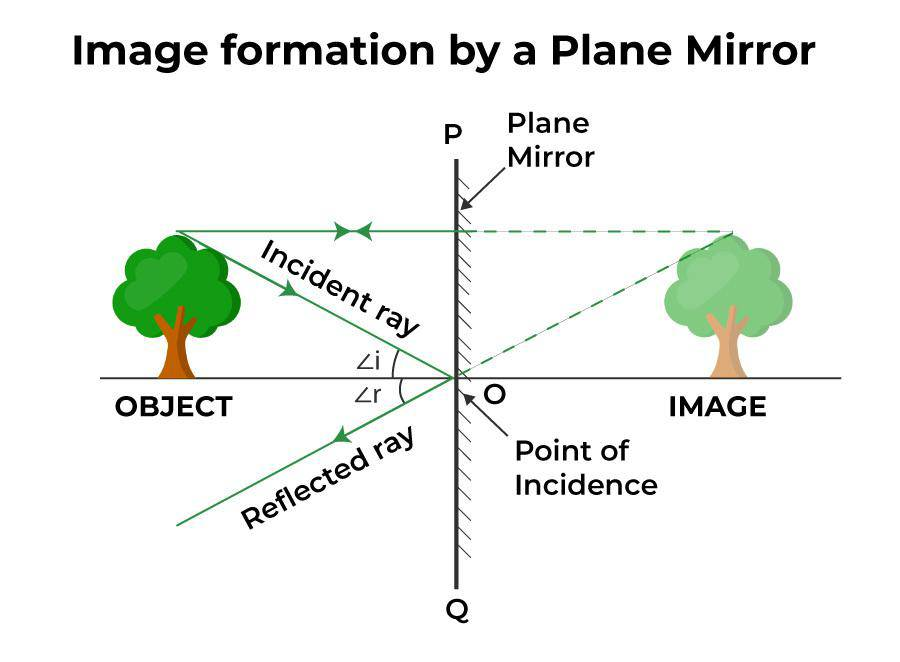GFG App
Open AppBrowser
Continue

Reflection or Reflection of light is the process of bouncing back light rays when it strikes the smooth and shiny reflecting surface. It is due to the reflection of light we are able to see ourselves in the Plane mirror. Light from our incident on the plane mirror gets reflected and so we are able to see our virtual image on the mirror. Reflection is produced by all the waves namely, Light waves, Electromagnetic waves, Sound waves, and waves that form on the water’s surface. The reflection of a light wave follows some set of rules known as the Laws of Reflection. There are two essential laws of reflection, according to which the angle of incidence and the angle of reflection made by the light wave on the smooth reflecting surface are equal. Also, the incident ray, normal, reflected ray, incident angle, and reflected angle, all lie on the same plane. In this article, we’ll learn What is a reflection of Light is? Reflection of light through the plane mirror, Laws and Types of reflection, along with frequently asked questions on the same.

## What is Reflection of Light?

The phenomenon, known as “reflection” or “reflection of light,” occurs when a light beam strikes any polished, smooth, or bright object and bounces back to our eyes. This phenomenon gives us a basis on which to view the environment. Light propagates in a straight line before, after, and during reflection. For example, the twinkling of stars or the light that a mirror reflects.Here is the list of important terminologies used in Reflection of Light as shown in the figure above:

• Normal: A perpendicular drawn to the reflecting surface at point M (known as the point of incidence) is called the normal to the reflecting surface.
• Incident Ray: A ray of light that falls on the reflecting surface from a light source or an object is called the incident ray.
• Reflected Ray: A ray of light that arises from the reflecting surface after reflection from it is called a reflected ray.
• Angle of Incidence: The angle between the incident ray and normal to the point of incidence on the reflecting surface is known as the incident angle or Bugle of incidence. It is denoted by ∠i.
• Angle of Reflection: The angle between the reflected ray and the normal to the point of incidence on the reflecting surface is known as the angle of reflection. It is denoted by ∠r.
• Principal axis: It is defined as a line that divides the two mediums or the reflecting surface is called the principal axis.

## Laws of Reflection

You must understand two necessary Laws of reflection after learning what it means. These rules can be used to calculate how the incident ray will reflect on different materials, such as a plane mirror, water, metal surfaces, etc. Here are the laws of reflection, as they apply to a plane mirror:• The incident ray, the reflected ray, and the normal all lie on the same plane.
• The angle of incidence (∠i) is always equal to the angle of reflection (∠r).

## Types of Reflection of Light

### Regular/Specular Reflection

A bright and sharp reflection, similar to those you see in mirrors, is referred to as a specular reflection. A uniform layer of a substance that is highly reflecting, such as powder, is applied to glass to create a mirror. This reflecting surface consistently reflects approximately all of the light that strikes it. The angles of reflection between different points don’t vary all that much. This indicates that the haze and blurring have been virtually gone.

### Diffused Reflection

In general, reflective surfaces other than mirrors have a highly rough surface. This might be the result of surface dirt or traces of wear and tear, including scratches and dents. Even the substance the surface is constructed of might be important at times. All of this causes the reflection to lose both brightness and quality.

When compared between sites on such uneven surfaces, the angle of reflection is completely random. When rays strike rough surfaces, they are reflected in radically different directions despite incidents at slightly different spots on the surface. We can perceive non-shiny objects because of a reflection type known as diffused reflection.## Reflection of light from the Plane Mirror

The light beams are reflected back when they strike the flat mirror. The angle of incidence and reflection are equal, according to the laws of reflection. Behind the plane, which is visible in the mirror, is where the image is obtained. A reflection on a plane mirror is the method by which a virtual, erect mirror image.The plane mirror always produces an erect, virtual image. The object’s size and the image’s size are both the same. The distance between the produced image and the mirror is the same as the position distance of the object. Images that are laterally inverted are obtained.

## FAQs on Reflection of Light

Question 1: What do mean by the reflection of light?

Reflection or Reflection of light is the process of bouncing back of light rays when it strikes the smooth and shiny reflecting surface.

Question 2: What are the two laws of reflection?

The two laws of reflection can be stated as:

1. The incident ray, the reflected ray, and the normal all lie on the same plane.
2. The angle of incidence (∠i) is always equal to the angle of reflection (∠r).

Question 3: Name the type of reflection that happens, when a clear and sharp reflection occurs.

Specular reflection.

Question 4: What type of image is formed by the plane mirror?